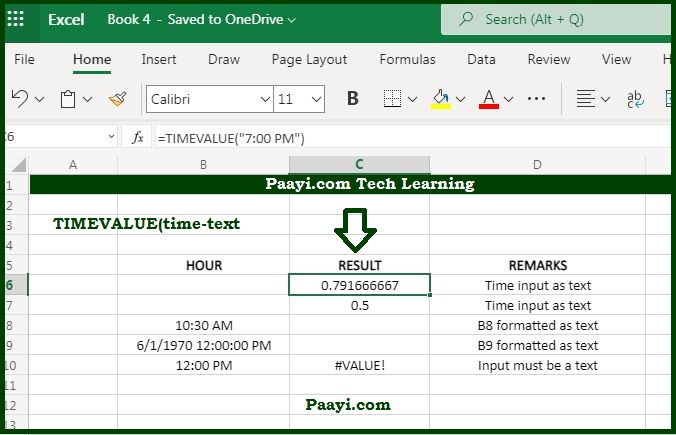# Learn How to Use Microsoft Excel TIMEVALUE Function

Written by | 0 Comments | 778 Views

In this article, you will learn how to use the Microsoft Excel TIMEVALUE function and its prime function in Microsoft Excel. You will also get to know the Microsoft Excel TIMEVALUE function return value and syntax with the help of some examples.

## Microsoft Excel TIMEVALUE Function

The prime purpose of the Microsoft Excel TIMEVALUE function is to get a valid time from a text string. So, with the TIMEVALUE function, you can convert the text into a proper Excel time. Let us understand this by the example, the formula = TIMEVALUE("9:00 AM") will return the 0.375, which is the numeric representation of the 9 AM in the Excel time system. In worksheets, the numeric time values are more useful than the text, as you can directly manipulate them with formulas and pivot tables.

### TIMEVALUE Function Return Value

The return value will be the decimal number corresponding to the specific time.

### TIMEVALUE Syntax

= TIMEVALUE(time-text)

Where:

• time-text: It is a date and/or time is a text format recognized by Excel.

## How to Use Microsoft Excel TIMEVALUE Function?The TIMEVALUE function creates a time in a serial number format from a date and/or time as per the Excel text format. TIMEVALUE function will return the value from 0 to 0.99988426, that represents - 12:00:00 AM to 11:59:59 PM.